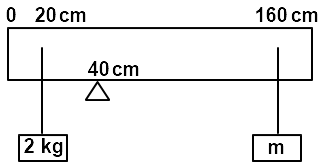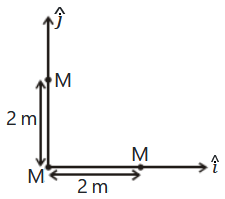From a circular ring of mass "M" and radius "R", an arc corresponding to a 90$°$ sector is removed. The moment of inertia of the remaining part of the ring about an axis passing through the centre of the ring and perpendicular to the plane of the ring is 'K' times $\text{'}{\mathrm{MR}}^{2}\text{'}$. The value of 'K' will be:

1. $\frac{1}{4}$

2. $\frac{1}{8}$

3. $\frac{3}{4}$

4. $\frac{7}{8}$

Subtopic:  Moment of Inertia |
To view explanation, please take trial in the course below.
NEET 2023 - Target Batch - Aryan Raj Singh
To view explanation, please take trial in the course below.
NEET 2023 - Target Batch - Aryan Raj Singh
Please attempt this question first.
Launched MCQ Practice Books

Prefer Books for Question Practice? Get NEETprep's Unique MCQ Books with Online Video/Text Solutions via Telegram Bot

A uniform rod of length 200 cm and mass 500 g is balanced on a wedge placed at 40 cm mark. A mass of 2 kg is suspended from the rod at 20 cm and another unknown mass 'm' is suspended from the rod at 160 cm mark as shown in the figure. Find the value of 'm' such that the rod is in equilibrium. (g=10 m/s2)1.

2.

3.

4.

Subtopic:  Torque |
To view explanation, please take trial in the course below.
NEET 2023 - Target Batch - Aryan Raj Singh
To view explanation, please take trial in the course below.
NEET 2023 - Target Batch - Aryan Raj Singh
Please attempt this question first.
Launched MCQ Practice Books

Prefer Books for Question Practice? Get NEETprep's Unique MCQ Books with Online Video/Text Solutions via Telegram Bot

Two particles of mass 5 kg and 10 kg respectively are attached to the two ends of a rigid rod of length 1m with negligible mass. The centre of mass of the system from the 5 kg particle is nearly at a distance of :

1. 50 cm

2. 67 cm

3. 80 cm

4. 33 cm

Subtopic:  Center of Mass |
To view explanation, please take trial in the course below.
NEET 2023 - Target Batch - Aryan Raj Singh
To view explanation, please take trial in the course below.
NEET 2023 - Target Batch - Aryan Raj Singh
Please attempt this question first.
Launched MCQ Practice Books

Prefer Books for Question Practice? Get NEETprep's Unique MCQ Books with Online Video/Text Solutions via Telegram Bot

Find the torque about the origin when a force 3$\stackrel{^}{\mathrm{j}}$ N acts on a particle whose position vector is 2$\stackrel{^}{\mathrm{k}}$ m.

1.  6$\stackrel{^}{\mathrm{j}}$ N m

2.  -6$\stackrel{^}{\mathrm{i}}$ N m

3.  6$\stackrel{^}{\mathrm{k}}$ N m

4. 6$\stackrel{^}{\mathrm{i}}$ N m

Subtopic:  Torque |
To view explanation, please take trial in the course below.
NEET 2023 - Target Batch - Aryan Raj Singh
To view explanation, please take trial in the course below.
NEET 2023 - Target Batch - Aryan Raj Singh
Please attempt this question first.
Launched MCQ Practice Books

Prefer Books for Question Practice? Get NEETprep's Unique MCQ Books with Online Video/Text Solutions via Telegram Bot

The angular speed of the wheel of a vehicle is increased from 360 rpm to 1200 rpm in 14 seconds. Its angular acceleration will be:

1.

2.

3.

4.

Subtopic:  Rotational Motion: Kinematics |
To view explanation, please take trial in the course below.
NEET 2023 - Target Batch - Aryan Raj Singh
To view explanation, please take trial in the course below.
NEET 2023 - Target Batch - Aryan Raj Singh
Please attempt this question first.
Launched MCQ Practice Books

Prefer Books for Question Practice? Get NEETprep's Unique MCQ Books with Online Video/Text Solutions via Telegram Bot

Three identical spheres, each of mass M, are placed at the corners of a right-angle triangle with mutually perpendicular sides equal to 2 m (see figure). Taking the point of intersection of the two mutually perpendicular sides as the origin, find the position vector of the centre of mass.1.  $2\left(\stackrel{^}{i}+\stackrel{^}{j}\right)$

2.  $\left(\stackrel{^}{i}+\stackrel{^}{j}\right)$

3.  $\frac{2}{3}\left(\stackrel{^}{i}+\stackrel{^}{j}\right)$

4.  $\frac{4}{3}\left(\stackrel{^}{i}+\stackrel{^}{j}\right)$

Subtopic:  Center of Mass |
To view explanation, please take trial in the course below.
NEET 2023 - Target Batch - Aryan Raj Singh
To view explanation, please take trial in the course below.
NEET 2023 - Target Batch - Aryan Raj Singh
Please attempt this question first.
Launched MCQ Practice Books

Prefer Books for Question Practice? Get NEETprep's Unique MCQ Books with Online Video/Text Solutions via Telegram Bot

A solid cylinder of mass 2 kg and radius 4 cm is rotating about its axis at the rate of 3 rpm. The torque required to stop after 2$\mathrm{\pi }$ revolutions is:

1.

2.

3.

4.

Subtopic:  Torque |
To view explanation, please take trial in the course below.
NEET 2023 - Target Batch - Aryan Raj Singh
To view explanation, please take trial in the course below.
NEET 2023 - Target Batch - Aryan Raj Singh
Please attempt this question first.
Launched MCQ Practice Books

Prefer Books for Question Practice? Get NEETprep's Unique MCQ Books with Online Video/Text Solutions via Telegram Bot

An object flying in the air with velocity $\left(20\stackrel{^}{i}+25\stackrel{^}{j}-12\stackrel{^}{k}\right)$ suddenly breaks into two pieces whose masses are in the ratio of 1:5. The smaller mass flies off with a velocity $\left(100\stackrel{^}{i}+35\stackrel{^}{j}+8\stackrel{^}{k}\right)$. The velocity of the larger piece will be:

1. $4\stackrel{^}{i}+23\stackrel{^}{j}-16\stackrel{^}{k}$

2. $-100\stackrel{^}{i}-35\stackrel{^}{j}-8\stackrel{^}{k}$

3. $20\stackrel{^}{i}+15\stackrel{^}{j}-80\stackrel{^}{k}$

4. $-20\stackrel{^}{i}-15\stackrel{^}{j}-80\stackrel{^}{k}$

Subtopic:  Linear Momentum |
To view explanation, please take trial in the course below.
NEET 2023 - Target Batch - Aryan Raj Singh
To view explanation, please take trial in the course below.
NEET 2023 - Target Batch - Aryan Raj Singh
Please attempt this question first.
Launched MCQ Practice Books

Prefer Books for Question Practice? Get NEETprep's Unique MCQ Books with Online Video/Text Solutions via Telegram Bot

A particle of mass 5m at rest suddenly breaks on its own into three fragments. Two fragments of mass m each move along mutually perpendicular directions with speed v each. The energy released during the process is :

1. $\frac{3}{5}m{v}^{2}$

2. $\frac{5}{3}m{v}^{2}$

3. $\frac{3}{3}m{v}^{2}$

4. $\frac{4}{3}m{v}^{2}$

Subtopic:  Linear Momentum |
To view explanation, please take trial in the course below.
NEET 2023 - Target Batch - Aryan Raj Singh
To view explanation, please take trial in the course below.
NEET 2023 - Target Batch - Aryan Raj Singh
Please attempt this question first.
Launched MCQ Practice Books

Prefer Books for Question Practice? Get NEETprep's Unique MCQ Books with Online Video/Text Solutions via Telegram Bot

A solid cylinder of mass 2 kg and radius 50 cm rolls up an inclined plane of angle of inclination ${30}^{°}$. The centre of mass of the cylinder has a speed of 4 m/s. The distance travelled by the cylinder on the inclined surface will be

1. 2.2 m

2. 1.6 m

3. 1.2 m

4. 2.4 m

Subtopic:  Rolling Motion |
To view explanation, please take trial in the course below.
NEET 2023 - Target Batch - Aryan Raj Singh
To view explanation, please take trial in the course below.
NEET 2023 - Target Batch - Aryan Raj Singh
Please attempt this question first.
Launched MCQ Practice Books

Prefer Books for Question Practice? Get NEETprep's Unique MCQ Books with Online Video/Text Solutions via Telegram Bot